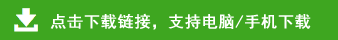# 人教版二年级数学下册口算+应用题综合练习题5套

34+31=     44+35=     31+52=
4×4=     12÷3=     19+30=
33+39=     98-32=     85-35=
3×4=     2÷1=     74-27=
87-36=     2×4=     16÷4=
79-33=     45+31=     62+27=
96-68=     21+63=     56+24=